1594188960

# Introduction

As a Data Scientist, there would be a time that we need to create a script or machine learning model to answer our question. Sometimes, there are many ways to solve our problem. Take an example of a classification problem; we might apply a probability-based model, tree-based model, or linear-based model. Initially, after EDA, we would choose the model with the assumption that filled by the data. Now, maybe we take a Random Forest model and want to train the model.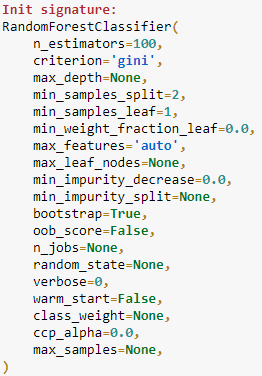Many of you would realise there are many parameters to tweak, just like in the picture beside. You then try each combination of the parameter until you find the ‘best’ parameter for your model, but it certainly would take a long time.

What I just explained in the passage above is what we called the optimization problem.

Formally, we could define the Optimization Problem as

“The problem of finding the best state according to the objective function.”

What we consider state would be varied as it depends on the context. It could be

• The parameter of the Random Forest Model;
• Chess position on the board;
• Neural network weight in the Deep Learning Model
• Train station placement order on the map.

The important thing here is that there are many possible states, but we want to find the **“best” **solution (or in the mathematical term objective functionfitness functioncost function, or loss function). For the optimization problem, we want to either maximize or minimize this function.

In the case of the optimization problem, because we compare each state to find the best function mathematically, it means the state should be a **numerical **value (or numerical vector). Optimization problems itself can be divided into continuous optimization, or discrete optimization depends on the variable.

# Mathematical Example

If you have a problem imagining it, let me show it by a simple mathematical function. Imagine if we have an objective function like below.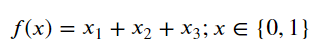where x is a vector consisting of the state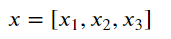Now, for example, we have an optimization problem where we want to maximize the Objective function or, in other words, have the maximum highest value as possible from the function. In our problem above, the function could only take a value between 0 or 1. now, we could have many combinations of x. It could be x = [0,0,0], or x = [0,1,1], etc. but we want to maximize the objective function. It means, the optimal solution would be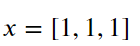where the function with f(x) set to the optimal solution is **f(x) = 3. **What I just explain is an example of the optimization problem where we want to have an objective function yield the maximum value.

In a real-world problem, the equation would never be that simple. It would often involve a complicated function and an abundant combination of state. It becomes a problem as we cannot optimize the objective function in a reasonable time.

#education #data-science #data #programming #data analysis #data analysis

## Buddha Community1605176864

## How to do Problem Solving as a Developer

In this video, I will be talking about problem-solving as a developer.

#problem solving skills #problem solving how to #problem solving strategies #problem solving #developer1599095520

## Complete Guide to Adam Optimization

In the 1940s, mathematical programming was synonymous with optimization. An optimization problem included an objective function that is to be maximized or minimized by choosing input values from an allowed set of values .

Nowadays, optimization is a very familiar term in AI. Specifically, in Deep Learning problems. And one of the most recommended optimization algorithms for Deep Learning problems is Adam.

Disclaimer: basic understanding of neural network optimization. Such as Gradient Descent and Stochastic Gradient Descent is preferred before reading.

### In this post, I will highlight the following points:

3. The Adam Algorithm for Stochastic Optimization
4. Visual Comparison Between Adam and Other Optimizers
5. Implementation
8. References

## 1. Definition of Adam Optimization

The Adam algorithm was first introduced in the paper Adam: A Method for Stochastic Optimization  by Diederik P. Kingma and Jimmy Ba. Adam is defined as “a method for efficient stochastic optimization that only requires first-order gradients with little memory requirement” . Okay, let’s breakdown this definition into two parts.

First, stochastic optimization is the process of optimizing an objective function in the presence of randomness. To understand this better let’s think of Stochastic Gradient Descent (SGD). SGD is a great optimizer when we have a lot of data and parameters. Because at each step SGD calculates an estimate of the gradient from a random subset of that data (mini-batch). Unlike Gradient Descent which considers the entire dataset at each step.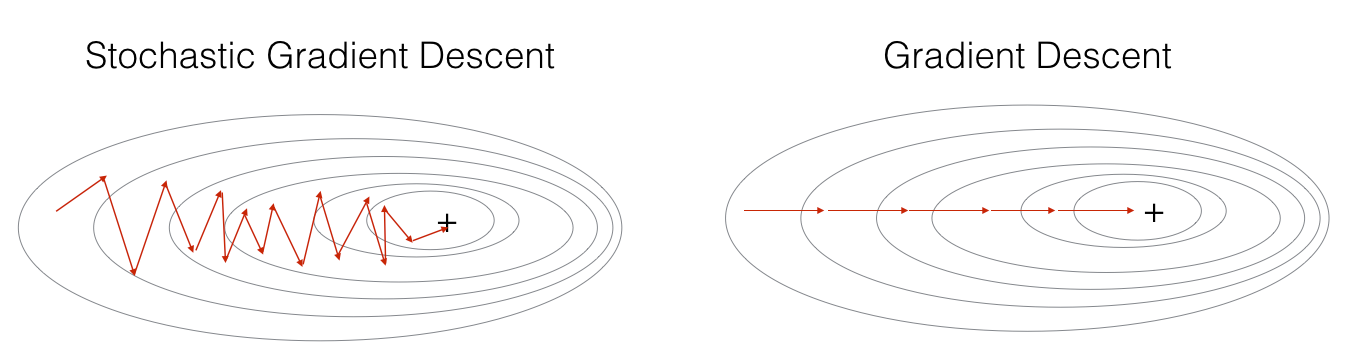1624496700

## Optimize Your Algorithms Tail Call Optimization

While writing code and algorithms you should consider tail call optimization (TCO).

### What is tail call optimization?

The tail call optimization is the fact of optimizing the recursive functions in order to avoid building up a tall call stack. You should as well know that some programming languages are doing tail call optimizations.

For example, Python and Java decided to don’t use TCO. While JavaScript allows to use TCO since ES2015-ES6.

Even if you know that your favorite language support natively TCO or not, I would definitely recommend you to assume that your compiler/interpreter will not do the work for you.

### How to do a tail call optimization?

There are two famous methods to do a tail call optimization and avoid tall call stacks.

### 1. Going bottom-up

As you know recursions are building up the call stack so if we avoid such recursions in our algorithms it will will allow us to save on the memory usage. This strategy is called the bottom-up (we start from the beginning, while a recursive algorithm starts from the end after building a stack and works backwards.)

Let’s take an example with the following code (top-down — recursive code):

``````function product1ToN(n) {
return (n > 1) ? (n * product1ToN(n-1)) : 1;
}
``````

As you can see this code has a problem: it builds up a call stack of size O(n), which makes our total memory cost O(n). This code makes us vulnerable to a stack overflow error, where the call stack gets too big and runs out of space.

In order to optimize our example we need to go bottom-down and remove the recursion:

``````function product1ToN(n) {
let result = 1;
for (let num = 1; num <= n; num++) {
result *= num;
}
return result;
}
``````

This time we are not stacking up our calls in the call stack, and we do use a O(1) space complexity(with a O(n) time complexity).

#memoization #programming #algorithms #optimization #optimize1603753200

## ML Optimization pt.1 - Gradient Descent with Python

So far in our journey through the Machine Learning universe, we covered several big topics. We investigated some regression algorithms, classification algorithms and algorithms that can be used for both types of problems (SVM**, **Decision Trees and Random Forest). Apart from that, we dipped our toes in unsupervised learning, saw how we can use this type of learning for clustering and learned about several clustering techniques.

We also talked about how to quantify machine learning model performance and how to improve it with regularization. In all these articles, we used Python for “from the scratch” implementations and libraries like TensorFlowPytorch and SciKit Learn. The word optimization popped out more than once in these articles, so in this and next article, we focus on optimization techniques which are an important part of the machine learning process.

In general, every machine learning algorithm is composed of three integral parts:

1. loss function.
2. Optimization criteria based on the loss function, like a cost function.
3. Optimization technique – this process leverages training data to find a solution for optimization criteria (cost function).

As you were able to see in previous articles, some algorithms were created intuitively and didn’t have optimization criteria in mind. In fact, mathematical explanations of why and how these algorithms work were done later. Some of these algorithms are Decision Trees and kNN. Other algorithms, which were developed later had this thing in mind beforehand. SVMis one example.

During the training, we change the parameters of our machine learning model to try and minimize the loss function. However, the question of how do you change those parameters arises. Also, by how much should we change them during training and when. To answer all these questions we use optimizers. They put all different parts of the machine learning algorithm together. So far we mentioned Gradient Decent as an optimization technique, but we haven’t explored it in more detail. In this article, we focus on that and we cover the grandfather of all optimization techniques and its variation. Note that these techniques are not machine learning algorithms. They are solvers of minimization problems in which the function to minimize has a gradient in most points of its domain.

### Dataset & Prerequisites

Data that we use in this article is the famous Boston Housing Dataset . This dataset is composed 14 features and contains information collected by the U.S Census Service concerning housing in the area of Boston Mass. It is a small dataset  with only 506 samples.For the purpose of this article, make sure that you have installed the following _Python _libraries:

• **NumPy **– Follow this guide if you need help with installation.
• **SciKit Learn **– Follow this guide if you need help with installation.
• Pandas – Follow this guide if you need help with installation.

Once installed make sure that you have imported all the necessary modules that are used in this tutorial.

``````import pandas as pd
import numpy as np
import matplotlib.pyplot as plt

from sklearn.model_selection import train_test_split
from sklearn.metrics import mean_squared_error
from sklearn.preprocessing import StandardScaler
from sklearn.linear_model import SGDRegressor
``````

Apart from that, it would be good to be at least familiar with the basics of linear algebracalculus and probability.

### Why do we use Optimizers?

Note that we also use simple Linear Regression in all examples. Due to the fact that we explore optimizationtechniques, we picked the easiest machine learning algorithm. You can see more details about Linear regression here. As a quick reminder the formula for linear regression goes like this: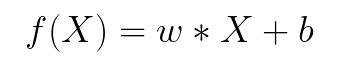where w and b are parameters of the machine learning algorithm. The entire point of the training process is to set the correct values to the w and b, so we get the desired output from the machine learning model. This means that we are trying to make the value of our error vector as small as possible, i.e. to find a global minimum of the cost function.

One way of solving this problem is to use calculus. We could compute derivatives and then use them to find places where is an extrema of the cost function. However, the cost function is not a function of one or a few variables; it is a function of all parameters of a machine learning algorithm, so these calculations will quickly grow into a monster. That is why we use these optimizers.

#ai #machine learning #python #artificaial inteligance #artificial intelligence #batch gradient descent #data science #datascience #deep learning #from scratch #gradient descent #machine learning #machine learning optimizers #ml optimization #optimizers #scikit learn #software #software craft #software craftsmanship #software development #stochastic gradient descent1624642980

## The Hitchhiker’s Guide to Optimization in Machine Learning

_NOTE: _For the sake of simplicity and better understanding, we‘ll restrict the scope of our discussion to supervised machine learning algorithms only.

Machine Learning is the ideal culmination of Applied Mathematics and Computer Science, where we train and use data-driven applications to run inferences on the available data. Generally speaking, for an ML task, the type of inference (i.e., the prediction that the model makes) varies on the basis of the problem statement and the type of data one is dealing with for the task at hand. However, in contrast to these dissimilarities, these algorithms tend to share some similarities as well, especially in the essence of how they operate.

Let’s try to understand the previous paragraph. Consider supervised ML algorithms as a superset. Now, we can go ahead and further divide this superset into smaller sub-groups based on the characteristics these algorithms share:

• Regression vs classification algorithms
• Parametric vs non-parametric algorithms
• Probabilistic vs non-probabilistic algorithms, etc.

Although setting these differences apart, if we observe the generalized representation of a supervised machine learning algorithm, it’s evident that these algorithms tend to work more or less in the same manner.

• Firstly, we have some labeled data, which can be broken down into the feature set X, and the corresponding label set Y.
• Then we have the model function, denoted by F, which is a mathematical function that maps the input feature set X_i t the output ŷ_i.

To put it in layman’s terms, every supervised ML algorithm involves passing as input to the model function F a feature set X_i, which the function F processes to generate an output ŷ_i.

However, this is just the inference (or testing) phase of a model, where theoritically, we are supposed to use the model to generate predictions on the data it has never seen before.

But what about “training” the model? Let’s have a look at it next.

#optimization #deep-learning #data-science #artificial-intelligence #machine-learning #optimization